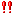catching < fweight fsize span bbox_x = "276" bbox_y = "1469" bbox_w = "128" bbox_h = "24" = "16" = "6" red = "81" green = "39" blue = "2" alpha = "255" > mas

exceptionally delicious trout in my < fweight fsize span bbox_x = "276" bbox_y = "1519" bbox_w = "272" bbox_h = "24" = "16" = "6" red = "81" green = "39" blue = "2" alpha = "255" >流しそうめんI keep getting various bell peppers, tomatoes, grapes, such as candy, not only < fweight fsize = span bbox_x = "276" bbox_y = "1690" bbox_w = "528" bbox_h = "49" "16" = "6" red = "81" green = "39" blue = "2" alpha = "255" > soumenMaybe < fweight fsize span bbox_x = "276" bbox_y = "1778" bbox_w = "112" bbox_h = "24" = "16" = "3" red = "81" green = "39" blue = "2" alpha = "255" > endgo < fweight fsize span bbox_x = "276" bbox_y = "1836" bbox_w = "128" bbox_h = "24" = "16" = "3" red = "81" green = "39" blue = "2" alpha = "255" > adult and< fweight fsize span bbox_x = "276" bbox_y = "1861" bbox_w = "272" bbox_h = "24" = "16" = "3" red = "81" green = "39" blue = "2" alpha = "255" > there are shallow waters where small children can play

from small camp < fweight fsize span bbox_x = "276" bbox_y = "1911" bbox_w = "480" bbox_h = "24" = "16" = "3" red = "81" green = "39" blue = "2" alpha = "255" > children's Association or Club, we accept reservations in groups.
< fweight fsize = span bbox_x = "276" bbox_y = "1936" bbox_w = "528" bbox_h = "49" "16" = "3" red = "81" green = "39" blue = "2" alpha = "255" > alone can see many smiles, so thinking along with the customer to suit your wishes and your budget, we will propose the plan!